Courses

# Using Influence Lines for Uniformly Distributed Load Civil Engineering (CE) Notes | EduRev

## Civil Engineering (CE) : Using Influence Lines for Uniformly Distributed Load Civil Engineering (CE) Notes | EduRev

The document Using Influence Lines for Uniformly Distributed Load Civil Engineering (CE) Notes | EduRev is a part of the Civil Engineering (CE) Course Structural Analysis I.
All you need of Civil Engineering (CE) at this link: Civil Engineering (CE)

Using Influence Lines for Uniformly Distributed Load

Consider the simply-supported beam AB in Figure 6.5, of which the portion CD is acted upon by a uniformly distributed load of intensity w/unit length . We want to find the value of a certain response function R under this loading and let us assume that we have already constructed the influence line of this response function. Let the ordinate of the influence line at a distance x from support A be FR (x) If we consider an elemental length dx of the beam at a distance x from A , the total force acting on this elemental length is wdx . Since dx is infinitesimal, we can consider this force to be a concentrated force acting at a distance x.The contribution of this concentrated force wdx to R is:

dR = (wdx)FR (x)

Therefore, the total effect of the distributed force from point C to D is: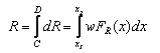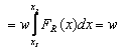(area under the influence line from C to D )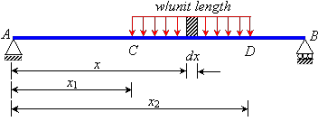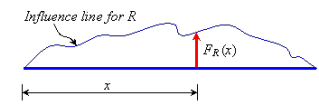Thus, we can obtain the response parameter by multiplying the intensity of the uniformly distributed load with the area under the influence line for the distance for which the load is acting. To illustrate, let us consider the uniformly distributed load on a simply supported beam (Figure 6.6). To find the vertical reaction at the left support, we can use the influence line for RA that we have obtained in Example 6.1. So we can calculate the reaction RA as:

RA = 2kN/m x {0.5(3/4 + 1/4} = 4kN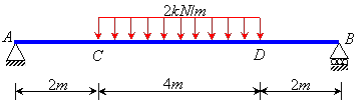Figure 6.6 Uniformly distributed load acting on a beam

Similarly, we can find any other response function for a uniformly distributed loading using their influence lines as well.

For non-uniformly distributed loading, the intensity w is not constant through the length of the distributed load. We can still use the integration formulation: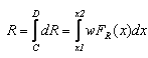However, we cannot take the intensity w outside the integral, as it is a function of x.

Offer running on EduRev: Apply code STAYHOME200 to get INR 200 off on our premium plan EduRev Infinity!

,

,

,

,

,

,

,

,

,

,

,

,

,

,

,

,

,

,

,

,

,

;